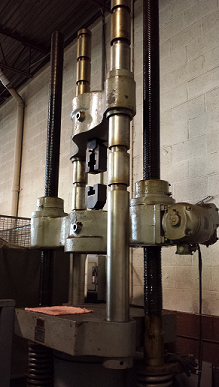In the fastener world, you’ll often hear terms like proof load, yield strength, and tensile strength tossed around when referring to the strength of a given fastener. For those unfamiliar with the precise meanings of these terms, I thought I’d devote a blog post to help define them and their relation to one another.

Proof load, yield strength, and tensile strength are numbers set by a standard that a fastener must meet in order to qualify as a certain grade or property class. All three numbers are set as minimum (and occasionally maximum) values. For example, according to ASTM A354, in order for a ½-13 bolt to qualify as grade BD, it must have a minimum proof load of 17,050 pounds-force (lbf), a minimum yield strength of 18,500 lbf, and a minimum tensile strength of 21,300 lbf. Not all standards specify requirements for all three tests. Yield strength and proof load are similar tests, so yield strength requirements are often omitted in favor of proof load requirements, as in SAE J429.

Before I can talk about individual terms, I should talk a bit about the kind of fastener strength involved here. All three terms involve the load that a threaded fastener can hold when pulled perpendicularly from the head. So, like this:In order to test this force, we use the tensile machine in our lab, which looks like this:As you can sort-of see, the fastener is fed into the slot in the middle. The machine then exerts a vertical force on the part. The machine measures the force as the part holds, distends, or breaks, depending on the test. To get an idea how each test works, read on.

Proof load is an amount of force that a fastener must be able to withstand without permanently deforming. So, to use the example above, in order to pass the proof load test set by ASTM A354, a ½-13 bolt must be able to hold a load of at least 17,050 lbf for a minimum of ten seconds without permanently elongating. The length of the part is measured before and after the proof load test to ensure compliance.

## What Is Yield Strength?

Yield strength is the load that is carried at the point where a fastener permanently deforms. When subjected to enough force, steel will begin to stretch. If the amount of force is low enough, the steel will elastically return to its original shape when the force is removed. At the yield point, the force becomes strong enough that the steel will stretch and not return to its original shape. This amount of force is the yield strength.

To test yield strength in our example, you would put our ½-13 bolt into the tensile machine, stretch the part until it distends, and calculate the force at the point of yield. In this case, the force would need to be a minimum of 18,500 lbf for the part to pass. The actual process of determining the force at the point of yield is rather engineer-y and involves graphs. If you would like to see it spelled out, check out ASTM F606.

## What Is Tensile Strength?

A fastener’s tensile strength, or ultimate tensile strength, is the force at which the fastener fractures. To test tensile strength, we use a wedge tensile test, where a wedge is placed under the head of the fastener and force is applied until the fastener breaks. A wedge tensile test looks like this:The wedge is used because it puts extra stress on the junction of the head and the body of the fastener. This ensures the absolute integrity of this junction. If the fastener breaks at a force greater than the minimum tensile requirement, the fastener has passed the tensile test. However, the break must not occur at the junction of the head and the body of the fastener. If the break does occur here, the fastener has failed tensile, regardless of the force at which the break occurred.

So to summarize, proof load is a load that can be held without permanent deformation. It is the lowest force of the three forces that we are discussing. Yield strength is the force exerted at which a fastener permanently deforms. Yield strength is a greater force than proof load. Finally, tensile strength is the force at which a fastener will break. It is the strongest of the three forces.